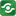Calculating Compound Interest@import url(http://www.google.com/cse/api/branding.css);CSGNetwork Custom SearchCSGNetwork Snippets Calculators Top Free Apps Home Back

Right click this window and select "view source" in order to copy the source for this script.

Calculating Compound Interest
First, the variables:

FV = future value
A = one-time investment (not for annuities)
p = investment per compound period
i = interest rate
c = number of compound periods per year
n = number of compound periods

To get p, take the target amount to invest each month, multiply it by 12 to get a yearly investment amount, then divide by c to get the investment per compound period. To get n, take the number of years to invest and multiply it by c to get the number of compound periods.

Simple compound interest with one-time investments... This is the formula that will present the future value (FV) of an investment after n years if we invest A at i interest compounded c times per year:

FV = A (1 + i/c)(n)

Required current investment (A) to have FV in the future if the i interest is compounded c times per year for n years:

FV
A = -----------
(1 + i/c)n

The time period (n) to have FV in the future if the initial investment A at i interest compounded c times per year:

ln(FV) - ln(A)
n = ------------------
ln(c + i) - ln(c)

NOTE: ln is the natural logarithm function.

 Finding the future value of a one-time investment Investment: Interest rate: number of times compounded each year: Number of years: Result:

 Finding the one-time investment neededto reach a desired future value Desired future value: Interest rate: Number of times compounded each year: Number of years: Result:

 Finding the number of years it will take toreach a desired future value Desired future value: Investment: Interest rate: Number of times compounded each year: Result:

Annuities are similar (but not identical) to one-time investments in all respects, except that you invest at regular intervals instead of just a one-time sum of money. For instance, investing \$150.00 per month in a mutual fund.

This formula presents how much we will have (FV) after n years if we invest p per compound period at i interest compounded c times per year:

p [(1 + i/c)n - 1]
FV = --------------------
(i/c)

How much is required per month (p) to reach \$1 million (FV) at i interest compounded c times per year for n years?

FVi
p = -------------------
c [(1 + i/c)n - 1]

How long is required (n) to reach \$1 million (FV) if p monthly investments at i interest compounded c times per year:

ln(FVi + cp) - ln(cp)
n = -------------------------
ln(c + i) - ln(c)

NOTE: ln is the natural logarithm function.

Annuities:

 Finding the future value of an annuity Investment per month: Interest rate: Number of times compounded each year: Number of years: Result:

 Finding the investment per month needed in anannuity to reach a desired future value Desired future value: Interest rate: Number of times compounded each year: Number of years: Result:

 Finding the number of years it will take to reacha desired future value in an annuity Desired future value: Investment per month: Interest rate: Number of times compounded each year: Result: